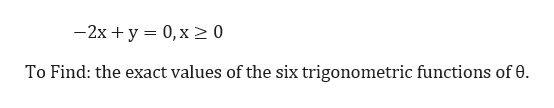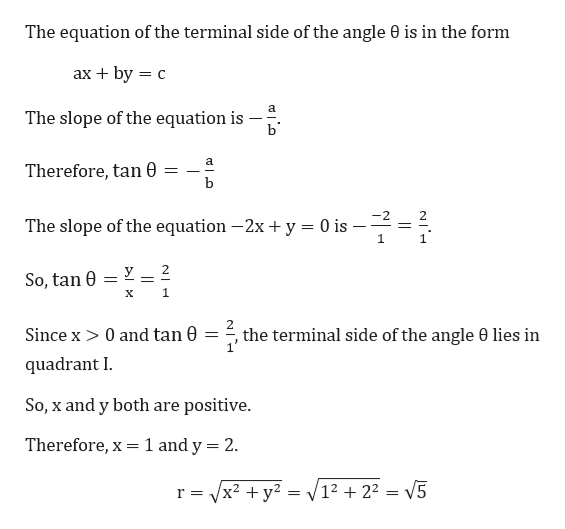# The equation, with a restriction on x, is the terminal side the of an angle θ in standard position. -2x + y = 0, x ≥ 0give the exact values of the six trigonometric functions of θ.

Question
85 views

The equation, with a restriction on x, is the terminal side the of an angle θ in standard position.

-2x + y = 0, x ≥ 0

give the exact values of the six trigonometric functions of θ.

check_circle

Step 1

Given the equation with a restriction on x, is the terminal side of an angle in standard position.help_outlineImage Transcriptionclose-2x+ y 0,x2 0 To Find: the exact values of the six trigonometric functions of 0 fullscreen
Step 2

We begin with comparing the given equ...help_outlineImage TranscriptioncloseThe equation of the terminal side of the angle 0 is in the form ах + by 3D с The slope of the equation is b Therefore, tan b The slope of the equation -2x + y = 0 is 1 _ 2 So, tan 0 1 х Since x> 0 and tan 0 = =, the terminal side of the angle 0 lies in quadrant I So, x and y both are positive. 1 and y 2 Therefore, x V12 +22 = V5 r Vx2 y2 fullscreen

### Want to see the full answer?

See Solution

#### Want to see this answer and more?

Solutions are written by subject experts who are available 24/7. Questions are typically answered within 1 hour.*

See Solution
*Response times may vary by subject and question.
Tagged in

### Trigonometric Ratios